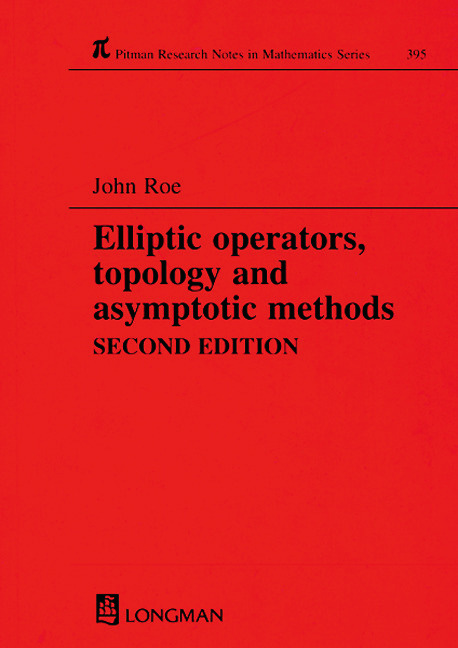# Elliptic Operators, Topology, and Asymptotic Methods

## 2nd Edition

Chapman and Hall/CRC

209 pages

Paperback: 9780582325029
pub: 1999-01-06
\$120.00
x
Hardback: 9781138417670
pub: 2017-08-15
\$205.00
x
eBook (VitalSource) : 9780429159763
pub: 2013-12-19
from \$57.50

FREE Standard Shipping!

### Description

Ten years after publication of the popular first edition of this volume, the index theorem continues to stand as a central result of modern mathematics-one of the most important foci for the interaction of topology, geometry, and analysis. Retaining its concise presentation but offering streamlined analyses and expanded coverage of important examples and applications, Elliptic Operators, Topology, and Asymptotic Methods, Second Edition introduces the ideas surrounding the heat equation proof of the Atiyah-Singer index theorem.

The author builds towards proof of the Lefschetz formula and the full index theorem with four chapters of geometry, five chapters of analysis, and four chapters of topology. The topics addressed include Hodge theory, Weyl's theorem on the distribution of the eigenvalues of the Laplacian, the asymptotic expansion for the heat kernel, and the index theorem for Dirac-type operators using Getzler's direct method. As a "dessert," the final two chapters offer discussion of Witten's analytic approach to the Morse inequalities and the L2-index theorem of Atiyah for Galois coverings.

The text assumes some background in differential geometry and functional analysis. With the partial differential equation theory developed within the text and the exercises in each chapter, Elliptic Operators, Topology, and Asymptotic Methods becomes the ideal vehicle for self-study or coursework. Mathematicians, researchers, and physicists working with index theory or supersymmetry will find it a concise but wide-ranging introduction to this important and intriguing field.

Resumé of Riemannian Geometry

Connections

Riemannian Geometry

Differential Forms

Exercises

Connection, Curvature, and Characteristic Classes

Principal Bundles and their Connections

Characteristic Classes

Genera

Notes

Exercises

Clifford Algebras and Dirac Operators

Clifford Bundles and Dirac Operators

Clifford Bundles and Curvature

Examples of Clifford Bundles

Notes

Exercises

The Spin Groups

The Clifford Algebra as a Superalgebra

Groups of Invertibles in the Clifford Algebra

Representation Theory of the Clifford Algebra

Spin Structures on Manifolds

Spin Bundles and Characteristic Classes

The Complex Spin Group

Notes

Exercises

Analytic Properties of Dirac Operators

Sobolev Spaces

Analysis of the Dirac Operator

The Functional Calculus

Notes

Exercises

Hodge Theory

Notes

Exercises

The Heat and Wave Equations

Existence and Uniqueness Theorems

The Asymptotic Expansion for the Heat Kernel

Finite Propagation Speed for the Wave Equation

Notes

Exercises

Traces and Eigenvalue Asymptotics

Eigenvalue Growth

Trace-Class Operators

Weyl's Asymptotic Formula

Notes

Exercises

Some Non-Compact Manifolds

The Harmonic Oscillator

Witten's Perturbation of the de Rham Complex

Functional Calculus on Open Manifolds

Notes

Exercises

The Lefschetz Formula

Lefschetz Numbers

The Fixed-Point Contributions

Notes

Exercises

The Index Problem

The Heat Equations and the Index Theorem

Notes

Exercises

The Getzler Calculus and the Local Index Theorem

Filtered Algebras and Symbols

Getzler Symbols

The Getzler Symbol of the Heat Kernel

The Exact Solution

The Index Theorem

Notes

Exercises

Applications of the Index Theorem

The Spinor Dirac Operator

The Signature Theorem

The Hirzebruch-Riemann-Roch Theorem

Local Index Theory

Notes

Exercises

Witten's Approach to Morse Theory

The Morse Inequalities

Morse Functions

The Contribution from the Circle Points

Notes

Atiyah's -Index Theorem

An Algebra of Smoothing Operators

Renormalized Dimensions an the Index Theorem

Notes

References